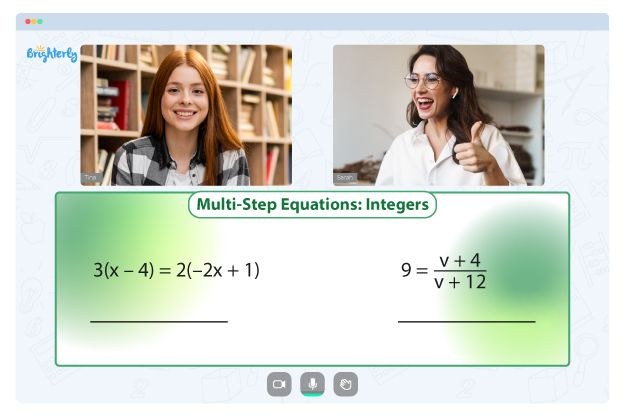# Term in Math – Definition, Examples, Practice Problems, FAQs

Welcome to Brighterly, where we transform math into a delightful adventure for children! In this captivating post, we will embark on an exciting journey through the realm of mathematical expressions, unraveling the mysteries of terms, factors, and coefficients. So buckle up and get ready to unleash the extraordinary power of math!

## What is Meant by Expression?

An expression is a combination of numbers, variables (like x, y, z), and mathematical operators (such as +, -, ×, ÷). Expressions do not contain an equal sign and do not represent a single value, but instead express a relationship between variables and constants. They are used to represent mathematical relationships and are the building blocks of equations and formulas.

For example, some expressions are:

• 3x + 5
• 2x² – 7x + 3
• 4y³ – y² + 2y – 1

Now that we have a basic understanding of expressions, let’s explore the components that make them up: terms, factors, and coefficients.

## What are Terms in an Expression?

A term is a single part of an expression, usually separated by addition (+) or subtraction (-) symbols. An expression can consist of one or more terms. In the expression 3x + 5, for example, there are two terms: 3x and 5.

Terms can be constants (like 5), variables (like x), or a combination of both, like 3x. Each term in an expression represents a unique mathematical relationship and helps us understand the overall behavior of the expression.

## What are the Factors of a Term?

Factors are the numbers or variables that are multiplied together to create a term. In the term 3x, the factors are 3 and x. In the term 2x², the factors are 2, x, and x (or 2 and x²).

Factors can help us simplify expressions and solve equations. For example, when we factor a quadratic expression, we can use the factors to find the solutions of the quadratic equation.

## What is a Coefficient in an Expression?

A coefficient is the numerical factor of a term containing a variable. It is the number that multiplies the variable. In the term 3x, the coefficient is 3, and in the term 2x², the coefficient is 2.

Coefficients help us understand the relationship between variables in an expression. They can represent a rate of change or a constant proportion, depending on the context of the problem.

## Solved Examples on Term

Let’s look at some examples to understand expressions, terms, factors, and coefficients better:

1. Identify the terms, factors, and coefficients in the expression 2x³ – 5x² + 7x – 3.
• Terms: 2x³, -5x², 7x, -3
• Factors:
• 2x³: 2, x, x, x (or 2 and x³)
• -5x²: -5, x, x (or -5 and x²)
• 7x: 7, x
• -3: -3
• Coefficients: 2, -5, 7

## Practice Questions on Term

Now it’s your turn! Try these practice questions to solidify your understanding:

1. Identify the terms, factors, and coefficients in the expression 4x²y – 3xy² + 2x – y.
2. What are the terms, factors, and coefficients in the expression 5x³ – 3x²y + 7x² – 8?
1. In the expression 2x⁴ – 6x³ + 9x² – 5, what are the terms, factors, and coefficients?

## Conclusion

Mastering expressions, terms, factors, and coefficients is paramount for laying a solid foundation in mathematics. These indispensable concepts empower us to dissect and manipulate mathematical relationships, crack equations, and streamline expressions. As you continue to practice and hone your skills, always remember that Brighterly is your steadfast companion, guiding you through every twist and turn on your exhilarating quest to conquer the world of math!

## Frequently Asked Questions on Term

### What is the difference between an expression and an equation?

An expression is a combination of numbers, variables, and mathematical operators that represents a mathematical relationship. An equation, on the other hand, is a statement that shows the equality between two expressions.

### What is the main difference between a term and a factor?

A term is a single part of an expression, separated by addition or subtraction symbols, while a factor is a number or variable that is multiplied together to create a term.

### Can a term have more than one coefficient?

No, a term can only have one coefficient. The coefficient is the numerical factor that multiplies the variable in the term.

Information Sources

For further reading and information on expressions, terms, factors, and coefficients, you can explore the following sources:

Problems with Equations?• Is your child finding it challenging to understand the concept of equations?
• An online tutor could be the solution.

Does your child struggle to master equations fundamentals? Try lessons with an online tutor.